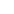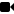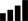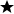# Online Class: Advanced Excel 2019

This advanced Excel 2019 course will show you how to do everything in Excel to make you an Excel Power User.

COURSE CLOSED

We're sorry, this course is no longer open for enrollment.

• 20
Lessons
• 36
Exams &
Assignments
• 16
Hours
average time
• 1.6
CEUs

## Course Description

Excel is one of the most powerful tools in Microsoft Office. You can import, query, analyze and manipulate data to create powerful reports that can be used by individuals and enterprise businesses alike. This course explores some of the more advanced features of Microsoft Excel to give you a more powerful experience when you need the most out of spreadsheet software.

• Outlines, Custom Views, and Templates
• Mathematical Functions
• Data Functions and Data Lookups
• The IF Function
• Manipulating Text
• Conditional Formatting
• Sparklines
• Importing, Cleaning and Validating Data
• Pivot Tables and Pivot Charts
• The What-If Analysis
• Goal Seek and Solver
• The Analysis ToolPak
• Using Get & Transform to Perform Queries
• Macros
• And Much More!
The course starts with functions and data lookups, as these components of Excel help the spreadsheet creator with advanced calculations and import functionality. We then cover conditional formatting for highlighting important cells based off of conditions. Sparklines are also covered so that the learner can get a quick data visualization of the information stored in each worksheet.

Pivot tables are one of the most beneficial tools in Excel, and this course covers pivot tables both basic and advanced features. It then moves on to pivot charts, which are graphs that can be used with pivot table data.

Analysis tools in Excel 2019 are some of the most advanced and useful to users that want to run complex reports. Excel has three What-If analysis tools that can be run on your data and provide reports on goals that give answers to your problems. The Solver and Analysis Toolpak are two add-ins that expand on these tools, and they are also covered in this course.

The final lessons cover data validation, troubleshooting, querying data and macros. These lessons provide ways to work with data and clean up unstructured information. This course fully covers advanced features in Excel so that you can create complex spreadsheets for business or individual use.

• Completely Online
• Self-Paced
• Printable Lessons
• Full HD Video• 6 Months to Complete
• Start Anytime
• PC & Mac Compatible
• Android & iOS Friendly
• Accredited CEUs## Course Lessons

#### Lesson 1: Outlines, Custom Views, and Templates

Microsoft includes numerous templates that you can choose from, and many times these templates include formulas and outlines that you can use to build a spreadsheet. Additional lesson topics: How to Use Excel Custom Views Like a Pro; Free Excel Templates; Microsoft Excel Inside Out 10 Total Points
•Lesson 1 Video
•Lesson discussions: Reasons for Taking this Course
•Complete Assignment: My Excel Experience
•Assessment: Lesson 1 Exam

#### Lesson 2: Mathematical Functions

Mathematical functions are a part of the spreadsheet creation process, but Excel has several that do the calculations for you. You can manually create your own functions or use the pre-created Excel functions. Additional lesson topics: SUM function; AVERAGE function; How to Use the RAND and RANDBETWEEN Functions in Excel; Excel functions alphabetical ; Countif function 11 Total Points
•Lesson 2 Video
•Complete: Lesson 2 Activity Assignment
•Assessment: Lesson 2 Exam

#### Lesson 3: Data Functions

Data functions (also called information functions) work with providing feedback about cell data based on the function used and the condition criteria. These functions include error information, numerical information or other information that tells you the type of data contained in a cell. Additional lesson topics: ERROR.TYPE function; Excel ISNUMBER Function; Excel ISREF Function; Excel ISBLANK Function; Excel TYPE Function; Excel ISERROR Function 11 Total Points
•Lesson 3 Video
•Complete: Lesson 3 Activity Assignment
•Assessment: Lesson 3 Exam

#### Lesson 4: Data Lookups

Excel has three ways to search for data within a spreadsheet: vertical, horizontal and both. This lesson will focus on these different search methods. Additional lesson topics: Excel VLOOKUP Function; HLOOKUP function 11 Total Points
•Lesson 4 Video
•Complete: Lesson 4 Activity Assignment
•Assessment: Lesson 4 Exam

#### Lesson 5: The IF Function

This lesson will focus on how to use the IF Function. An IF statement returns "true" or "false" as a result, and you can display data based on this result. Within an IF statement, you can have other functions and formulas to create complex, embedded calculations that display data based on evaluation of several rows and columns in your spreadsheet. Additional lesson topics: Excel IF Function; Conditional Formulas in Excel and Tricks Using the IF Function in Excel; Excel logical formulas: Several simple IF statements to get started; Excel Nested IF statement: examples, best practices and alternatives 11 Total Points
•Lesson 5 Video
•Complete: Lesson 5 Activity Assignment
•Assessment: Lesson 5 Exam

#### Lesson 6: Manipulating Text

Excel has numerous numerical and logical functions, but text functions used to manipulate text are often used. These functions are usually used in combination with formatting functions to automatically and dynamically stylize the way information displays in a cell. Additional lesson topics: Excel FIND Function; Functions LEFT – RIGHT – MID; Change the case of text; Excel SUBSTITUTE Function 11 Total Points
•Lesson 6 Video
•Complete: Lesson 6 Activity Assignment
•Assessment: Lesson 6 Exam

#### Lesson 7: Exploring Conditional Formatting

With conditional formatting, you can change colors and styles of a cell based on the results of a formula. Formulas return values, and Excel's conditional formatting features will take these results and change a cell's style based on a true or false value. Additional lesson topics: Analyze and format in Excel; Microsoft Excel : Conditional Formatting 11 Total Points
•Lesson 7 Video
•Complete: Lesson 7 Activity Assignment
•Assessment: Lesson 7 Exam

#### Lesson 8: Working with Sparklines

One reason to use Excel over other spreadsheet software is for its numerous graphing and visual data representation tools. Sparklines are one graphing tool that allows you to display a visual representation of data within one cell. Additional lesson topics: Use formulas with conditional formatting; Use sparklines to show data trends; Excel Sparklines – A Complete Guide with Examples 11 Total Points
•Lesson 8 Video
•Complete: Lesson 8 Activity Assignment
•Assessment: Lesson 8 Exam

#### Lesson 9: Linking To and Consolidating Worksheets

Excel has functions that let you retrieve and consolidate data across multiple sheets and multiple workbooks. These features let you calculate data in different workbooks and worksheets without the need to copy and paste data to the same location. Additional lesson topics: Consolidate data in Excel and merge multiple sheets into one worksheet 11 Total Points
•Lesson 9 Video
•Complete: Lesson 9 Activity Assignment
•Assessment: Lesson 9 Exam

#### Lesson 10: Importing and Cleaning Data

This lesson will focus on how to import and use many forms of data. Additional lesson topics: How to use Goal Seek in Excel for What-If analysis; Microsoft Excel: How to use Scenario Manager 11 Total Points
•Lesson 10 Video
•Complete: Lesson 10 Activity Assignment
•Assessment: Lesson 10 Exam

#### Lesson 11: Pivot Tables

Pivot tables have a bad reputation of being complex and difficult to understand, but recent versions of Excel have made working with pivot tables much easier. Additional lesson topics: How to Create A New Pivot Table With The Excel Quick Analysis Tool; Create a PivotTable to analyze worksheet data; Summarizing Text Data With Pivot Tables 11 Total Points
•Lesson 11 Video
•Complete: Lesson 11 Activity Assignment
•Assessment: Lesson 11 Exam

#### Lesson 12: Continuing to Work with Pivot Tables

This lesson will continue exploring various uses of Pivot Tables Additional lesson topics: Calculate values in a PivotTable; Calculated Field or Calculated Item; Use slicers to filter data; Create a timeline 10 Total Points
•Lesson 12 Video
•Assessment: Lesson 12 Exam

#### Lesson 13: Pivot Charts

A pivot chart displays data in various ways so that you can review and define the story that your information tells you about your business. A pivot chart complements this data by giving you a visual representation of the information in your pivot table. Additional lesson topics: Create a PivotChart; Add a data series to your chart; Change the layout or style of a chart 11 Total Points
•Lesson 13 Video
•Complete: Lesson 13 Activity Assignment
•Assessment: Lesson 13 Exam

#### Lesson 14: The What-if Analyses

In business, you must make projections and changes that could increase revenue. Before you make any changes, you should analyze data. A What-If analysis lets you create scenarios where you can ask a question from your data and get results based on the data and the scenario that you input. Additional lesson topics: Find Quick Answers With Excel Goal Seek; Excel Scenarios Create and Show 10 Total Points
•Lesson 14 Video
•Assessment: Lesson 14 Exam

#### Lesson 15: Goal Seek and Solver

The Goal Seek tool in Excel helps you find answers to possible outcomes. You control these outcomes using variables and values until you find a number that matches your goals. Additional lesson topics: How to Use Excel’s Goal Seek and Solver to Solve for Unknown Variables; Load the Solver Add-in in Excel; Define and solve a problem by using Solver 11 Total Points
•Lesson 15 Video
•Complete: Lesson 15 Activity Assignment
•Assessment: Lesson 15 Exam

#### Lesson 16: The Analysis ToolPak

The Solver tool offers ways to find solutions to your problems, but the Analysis Toolpak takes analysis a step further and lets you perform evaluations on complex statistical and engineering problems. Additional lesson topics: Use the Analysis ToolPak to perform complex data analysis; Load the Analysis ToolPak in Excel; The t-Test Tool 9 Total Points
•Lesson 16 Video
•Assessment: Lesson 16 Exam

#### Lesson 17: All About Data Validation

You can control the type of data entered into a cell by using Excel's data validation tools. These tools will block other formats and reduce the number of mistakes made when you rely on others to enter the right type of data into a record. Additional lesson topics: Apply data validation to cells; Display or hide circles around invalid data; How to Remove Duplicates In Excel : Eliminating Records With Duplicate Fields 11 Total Points
•Lesson 17 Video
•Complete: Lesson 17 Activity Assignment
•Assessment: Lesson 17 Exam

#### Lesson 18: Using Get & Transform to Perform Queries

The "Get & Transform" feature imports data from a third-party source and pours the data into a worksheet. This could be a dozen records or thousands of records. Additional lesson topics: Overview of Excel tables; Connect to an Excel table or range Power Query ; Create and format tables 10 Total Points
•Lesson 18 Video
•Assessment: Lesson 18 Exam

#### Lesson 19: Troubleshooting and Auditing in Excel 2019

Excel has some internal features that help you find dependent cells and troubleshoot issues. Additional lesson topics: Detecting and Correcting Errors In Excel Formulas 9 Total Points
•Lesson 19 Video
•Assessment: Lesson 19 Exam

#### Lesson 20: Exploring Macros in Excel 2019

Macros are useful for repetitive steps you perform on every spreadsheet. You can record macros in the main Excel interface or create highly complex automated macros in Microsoft's proprietary Visual Studio for Applications (VBA). 86 Total Points
•Lesson 20 Video
•Lesson discussions: End of Course Poll; Course Comments; Program Evaluation Follow-up Survey (End of Course)
•Complete: Lesson 20 Activity Assignment
•Assessment: Lesson 20 Exam
•Assessment: The Final Exam
287
Total Course Points

## Learning Outcomes

By successfully completing this course, students will be able to:
• Demonstrate working with outlines, custom views, and templates in Excel 2019.
• Demonstrate using mathematical functions.
• Demonstrate using data functions, data lookups, and the IF function.
• Demonstrate using conditional formatting.
• Demonstrate working Sparklines.
• Demonstrate linking to and consolidating worksheets.
• Describe importing and cleaning data.
• Demonstrate creating and using Pivot Tables and Pivot Charts.
• Demonstrate creating and using the What-if Analyses.
• Demonstrate creating and using the Goal Seek and Solver.
• Demonstrate creating and using the the Analysis ToolPak.
• Demonstrate creating and using the using get & transform to perform queries.
• Demonstrate mastery of lesson content at levels of 70% or higher.

## Student Testimonials

• "i found this course to be very thorough in explanation. The detail and explanation was very easy to follow and look back at." -- Patricia A.
• "Some parts were easier than others but I was able to learn many new things in Excel that I was not aware of previously. I look forward to using these in my job." -- Patti M.
• "This course was well put together and helped me grasp all concepts offered." -- David G.
• "Thank you for going the extra mile. Loved the course!" -- Sean B.
• "I think the course is very helpful. I learned a lot of things I did not know." -- Myrtal W.
• "Very helpful! Thank you." -- Kathleen P.
• "Excellent Course." -- Evelyn E.
• "This course if very helpful for an analyst." -- Brittany H.
• "Great! I learned a lot." -- Deborah H.